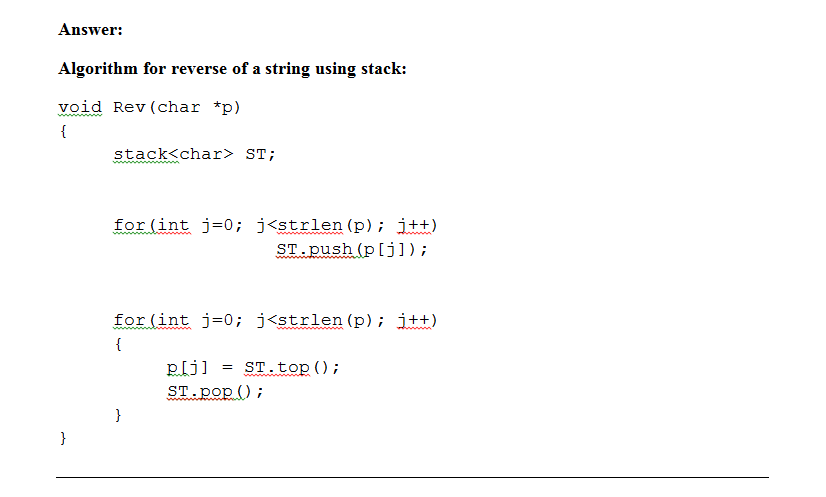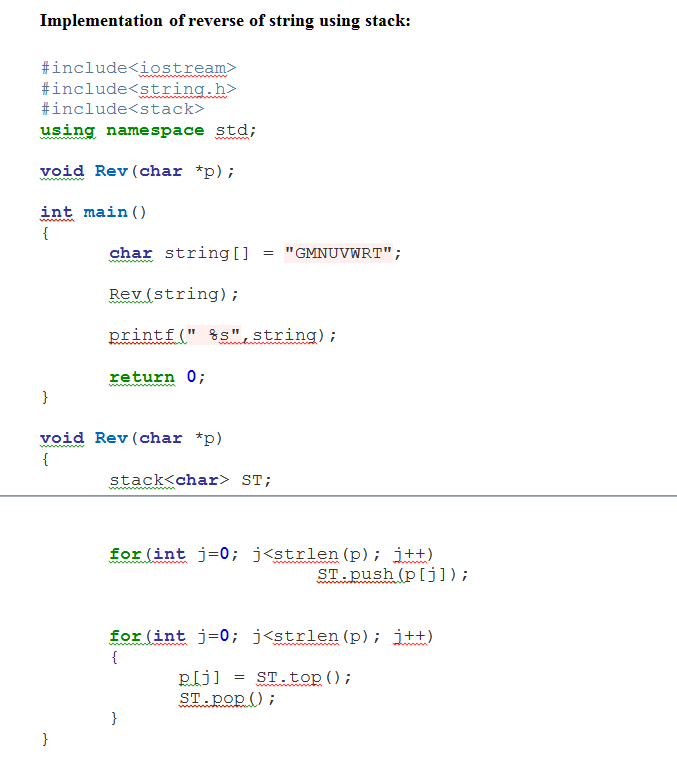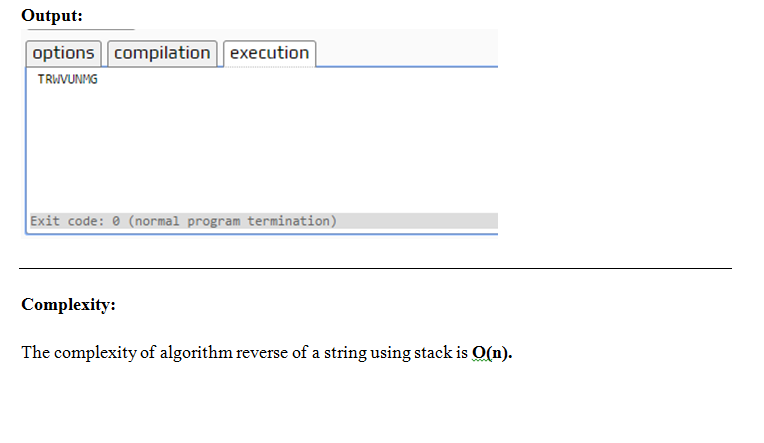# Answered! For the following problem use a Stack. Write an algorithm to reverse the string: "GMNUVWRT"…

For the following problem use a Stack.

Write an algorithm to reverse the string: “GMNUVWRT”

Don't use plagiarized sources. Get Your Custom Essay on
Answered! For the following problem use a Stack. Write an algorithm to reverse the string: "GMNUVWRT"…
GET AN ESSAY WRITTEN FOR YOU FROM AS LOW AS \$13/PAGE

Implement the algorithm using C++

FInd the complexity of the algorithm.Editable Code:

#include<iostream>
#include<string.h>
#include<stack>
using namespace std;

void Rev(char *p);

int main()
{
char string[] = “GMNUVWRT”;

Rev(string);

printf(” %s”,string);

return 0;
}

void Rev(char *p)
{
stack<char> ST;

for(int j=0; j<strlen(p); j++)
ST.push(p[j]);

for(int j=0; j<strlen(p); j++)
{
p[j] = ST.top();
ST.pop();
}
}